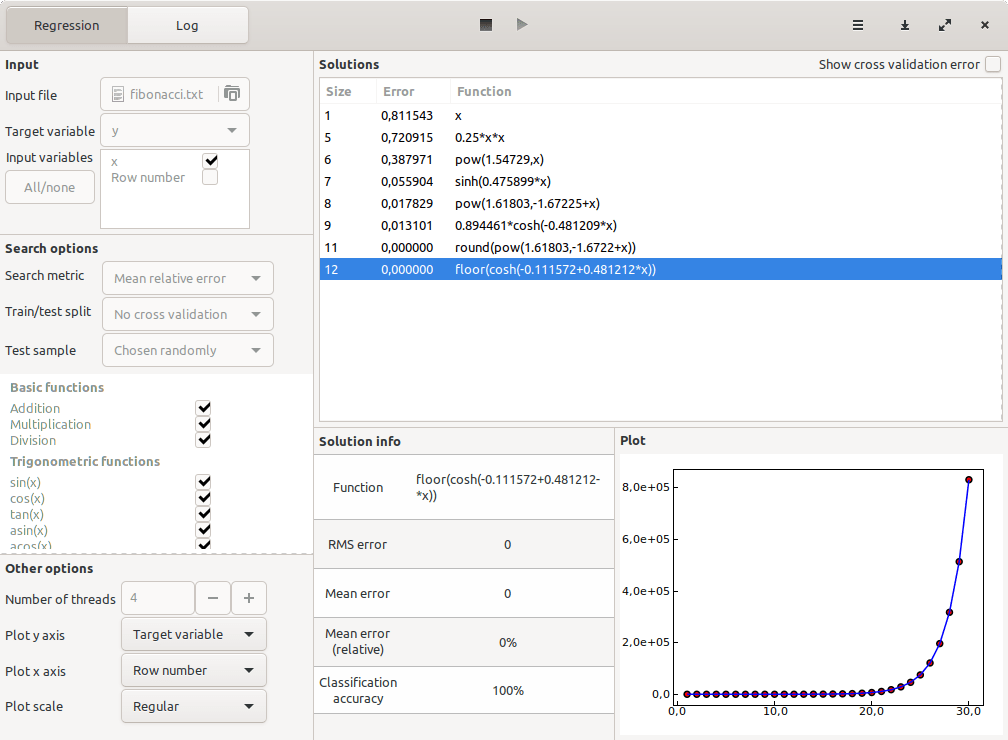# How to find a formula for the nth term of a sequence

Given a sequence of numbers, finding an explicit mathematical formula that computes the nth term of the sequence can be challenging, except in very special cases like arithmetic and geometric sequences.

In the general case, this task involves searching over the space of all mathematical formulas for the most appropriate one. A special technique exists that does just that: symbolic regression. Here we will introduce how it works, and use it to find a formula for the nth term in the Fibonacci sequence (A000045 in the OEIS) as an example.

### What symbolic regression is

Regression is the task of establishing a relationship between an output variable and one or more input variables. Symbolic regression solves this task by searching over the space of all possible mathematical formulas for the ones with the greatest accuracy, while trying to keep those formulas as simple as possible.

The technique starts from a set of base functions — for instance, sin(x), exp(x), addition, multiplication, etc. Then it tries to combine those base functions in various ways using an optimization algorithm, keeping track of the most accurate ones found so far.

An important point in symbolic regression is simplicity. It is easy to find a polynomial that will fit any sequence of numbers with perfect accuracy, but that does not really tell you anything since the number of free parameters in the model is the same as the number of input variables. For this reason, a symbolic regression procedure will discard a larger formula if it finds a smaller one that performs just as well.

### Finding the nth Fibonacci term

Now let’s show how symbolic regression can be used in practice by trying to find a formula for the Fibonacci sequence using the desktop symbolic regression software TuringBot. The first two terms of the sequence are 1 and 1, and every next term is defined as the sum of the previous two terms. Its first terms are the following, where the first column is the index:

```1 1
2 1
3 2
4 3
5 5
6 8
7 13
8 21
9 34
10 55```

A list of the first 30 terms can be found on this file: fibonacci.txt.

TuringBot takes as input TXT or CSV files with one variable per column and efficiently finds formulas that connect those variables. This is how it looks like after we load fibonacci.txt and run the optimization:Finding a formula for the nth Fibonacci term with TuringBot.

The software finds not only a single formula, but the best formulas of all possible complexities. A larger formula is only shown if it performs better than all simpler alternatives. In this case, the last formula turned out to predict with perfect accuracy every single one of the first 30 Fibonacci terms. The formula is the following:

`f(x) = floor(cosh(-0.111572+0.481212*x))`

Clearly a very elegant solution. The same procedure can be used to find a formula for the nth term of any other sequence (if it exists).

### Conclusion

In this tutorial, we have seen how the symbolic regression software TuringBot can be used to find a closed-form expression for the nth term in a sequence of numbers. We found a very short formula for the Fibonacci sequence by simply writing it into a text file with one number per row and loading this file into the software.

If you are interested in trying TuringBot your own data, you can download it from the official website. It is available for both Windows and Linux.